# User Reference:LPFilter

## Function

The LPFilter is a simple single-pole temporal low pass filter with a time constant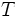$T$ (given in units of a sample's duration), a sequence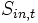$S_{in,t}$ as input and a sequence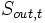$S_{out,t}$ as output (where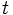$t$ is a sample index proportional to time), and obeying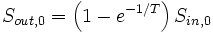$S_{out, 0} = \left( 1-e^{-1/T} \right) S_{in, 0}$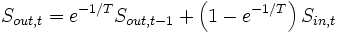$S_{out, t} = e^{-1/T} S_{out, t-1} + \left( 1-e^{-1/T} \right) S_{in, t}$

Typically, the LPFilter would be used to remove high-frequency noise from the Classifier's output.

## Parameters

### LPTimeConstant

The filter's time constant in units of sample blocks, or in seconds if followed with an "s".

Consistent with its mathematical limit, a value of
LPTimeConstant= 0
disables the LPFilter.

None.